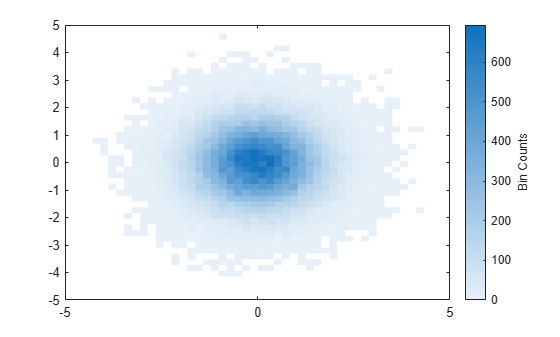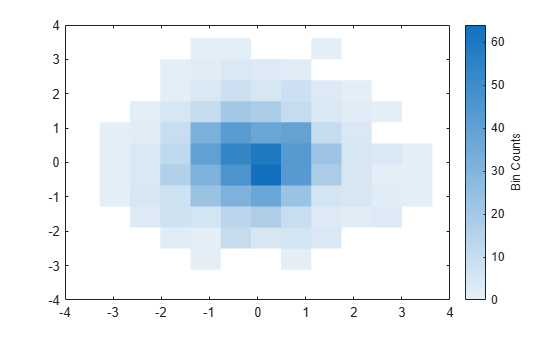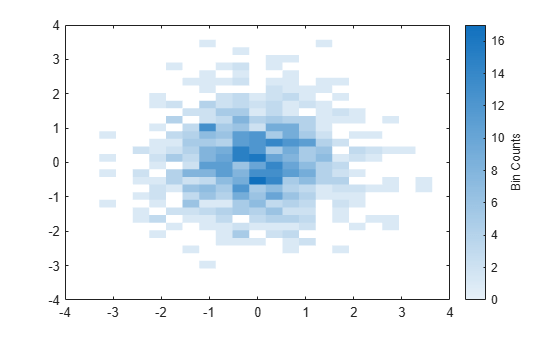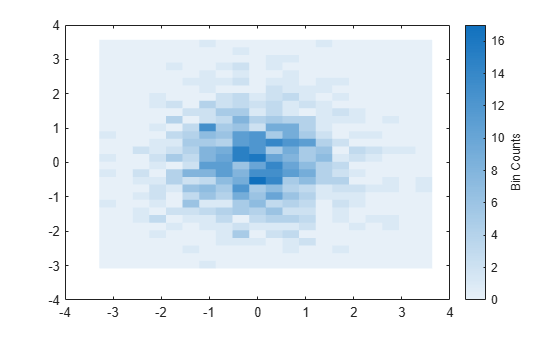# binscatter

## 语法

``binscatter(x,y)``
``binscatter(x,y,N)``
``binscatter(___,Name,Value)``
``binscatter(ax,___)``
``h = binscatter(___)``

## 说明

``binscatter(x,y)` 显示向量 `x` 和 `y` 的分 bin 散点图。分 bin 散点图将数据空间分成多个矩形 bin，并用不同颜色显示每个 bin 中的数据点数。放大绘图时，bin 的尺寸会自动调整，以显示更清晰的分辨率。`

``binscatter(x,y,N)` 指定要使用的 bin 数。`N` 可以是标量或二元素向量 `[Nx Ny]`。如果 `N` 是标量，则 `Nx` 和 `Ny` 都设置为标量值。每个维度中的最大 bin 数为 250。`
``binscatter(___,Name,Value)` 使用一个或多个名称-值对组参数指定属性值。例如，您可以将 `'ShowEmptyBins'` 指定为 `'on'`，以便对无数据点的区域进行着色。有关属性的完整列表，请参阅 Binscatter 属性。可将此选项与上述语法中的任何输入参数组合一起使用。`
``binscatter(ax,___)` 将图形绘制到 `ax` 指定的坐标区中，而不是当前坐标区 (`gca`) 中。`ax` 输入可以位于上述语法中的任何输入参数组合之前。`

``h = binscatter(___)` 返回 `Binscatter` 对象。使用此对象可检查和调整分 bin 散点图的属性。`

## 示例

xy 维上生成随机数，并创建分 bin 散点图。`binscatter` 函数会自动选择合适的 bin 数量，以便涵盖数据中的值范围。

```x = randn(1e6,1); y = 2*x + randn(1e6,1); binscatter(x,y)``````rng default % for reproducibility x = randn(1e4,1); y = randn(1e4,1); h = binscatter(x,y,[30 10]);````counts = h.Values;`

```x = randn(1e5,1); y = randn(1e5,1); binscatter(x,y)````colormap(gca,'parula')````x = randn(1000,1); y = randn(1000,1); h = binscatter(x,y)``````h = Binscatter with properties: NumBins: [11 11] XBinEdges: [-3.2764 -2.6485 -2.0206 -1.3927 -0.7648 -0.1369 0.4910 ... ] YBinEdges: [-3.1155 -2.5034 -1.8914 -1.2794 -0.6674 -0.0553 0.5567 ... ] Values: [11x11 double] XLimits: [-3.2764 3.6305] YLimits: [-3.1155 3.6168] FaceAlpha: 1 Show all properties ```

`h.NumBins = [20 30];``h.ShowEmptyBins = 'on';````xlim(gca,h.XLimits); ylim(gca,h.YLimits); h.XLimits = [-1 1];```## 输入参数

bin 数量，指定为标量或二元素向量 `[Nx Ny]`

• 如果 `N` 是一个二元素向量 `[Nx Ny]`，则 `binscatter` 在 x 维度上划分 `Nx` 个 bin，在 y 维度上划分 `Ny` 个 bin。

• 如果 `N` 是标量，则 `Nx``Ny` 都设置为标量值。

### 名称-值参数

x 维度的数据范围，指定为二元素向量 `[Xmin Xmax]`

`binscatter` 仅显示落在指定数据范围 ${X}_{\mathrm{min}}\le X\le {X}_{\mathrm{max}}$（含边界值）内的数据点。

y 维度的数据范围，指定为二元素向量 `[Ymin Ymax]`

`binscatter` 仅显示落在指定数据范围 ${Y}_{\mathrm{min}}\le Y\le {Y}_{\mathrm{max}}$（含边界值）内的数据点。

## 输出参数

`Binscatter` 对象。使用此对象可检查和调整绘图的属性。有关属性的完整列表，请参阅 Binscatter 属性

## 提示

• 当几个 bin 控制整个绘图时，将坐标区的 `ColorScale` 属性更改为 `'log'` 可以产生更好的 bin 着色。

```ax = gca; ax.ColorScale = 'log';```